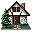Home Existing users log in here or new users sign up here. Kinematics Puzzles Mechanics Puzzles Electric Circuits Puzzles Magnetism Puzzles Kinematics Surprising Data Mechanics Surprising Data Electrostatics Surprising Data Fluids Surprising Data Optics Surprising Data

### Projectile Problem: Testing Experiment

#### Prior Knowledge

• Motion with constant speed
• Motion with constant acceleration
• Projectile motion

#### Description of the Experiment

A ball is launched at an angle of 30 degrees and then at an angle of 60 degrees. Make a prediction about how far the ball will fly in each case if the initial launch velocity is 4.8m/s in each case. (With an uncertainty of 0.1m/s.)

• Explain what models/explanations you are using to make your prediction.
• Make a list of assumptions you are making to make your prediction.
• How will each assumption affect your prediction?
• Make a prediction for how far the ball will travel at 30 degrees.
• Make a prediction for how far the ball will travel at 60 degrees.

Now watch the video, take data (there are two trials for each angle) and see if the results of the experiment match your prediction.